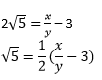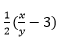Guru

# Prove that 3 + 2√5 + is irrational. Q.2

• 0

Another best question of irrational number of class 10th . How can we solve this question . Please give me some suggestion for this question. The best way for solving this question. Prove that 3 + 2√5 + is irrational.

Share

1. Let us assume 3 + 25 is rational.

Then we can find co-prime x and y (y ≠ 0) such that 3 + 2√5 = x/y

Rearranging, we get,Since, x and y are integers, thus,is a rational number.

Therefore, 5 is also a rational number. But this contradicts the fact that 5 is irrational.

So, we conclude that 3 + 25 is irrational.

• 0